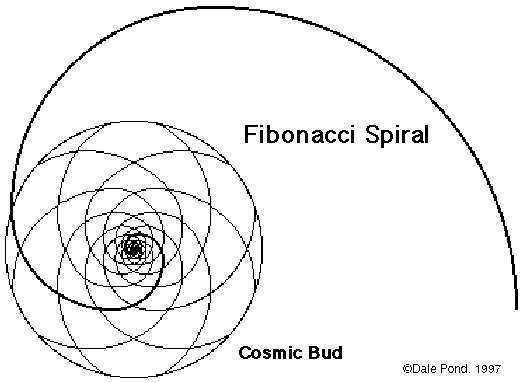# Fibonacci RelationshipsFibonacci Spiral

Fibonacci numbers constitute a special relativity or relationship between themselves and are an arithmetical progression that is additive and accumulative. Such functions become part of the next step in that going from 1+ to 2+ to 3+ to 4++ are steps that progress with the first quantity added to the next quantity and so on. This is a Fibonacci Progression of function of 1 + 2 = 3 and 2 + 3 = 4 (remembering these are steps and not numeric quantities). So the power accumulation (syntropy) spirals inward while the power dissipation (entropy) spirals outward in reverse Fibonacci series. The Russell Indig numbers are as the Fibonacci Series in that they add and accummulate in a specific and special relationship when syntropic. Of course the reverse is true when entropic. Being syntropic Fibonacci progressions are what life is.